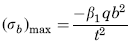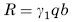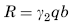Related Resources: calculators

Flat Rectangular Plate, Three Edges Fixed, One Edge (a) Simply Supported Loading Uniformly Over Entire Plate Stress and Reaction Loading Equation and Calculator.

Per. Roarks Formulas for Stress and Strain for flat plates with straight boundaries and constant thickness

Flat Rectangular plate, Three Edges Fixed, One Edge Loading Uniformly Over Entire Plate

 Rectangular plate; Three Edges FixedAt x = 0, z = 0
Stress and Reaction ForceReaction ForceStress and Reaction Force at x = 0, z = bStress and Reaction Force at x = ± a/2, z = bReaction ForceWhere used:
E = Modulus of Elasticity (lbs/in2)
q = Total load or force to Plate (lbs/in2)
v = Poisson’s ratio (assumed to be 0.3)
t = plate thickness, (in)
a = plate length, (in)
b = plate width, (in)
σb = stress, (lbs/in2)
R = Reaction Force (lbs/in)
β1,2,3 = Constant From Table A
γ1,2 = Constant From Table A

Table A

 a/b 0.25 0.5 0.75 1 1.5 2 3 β1 0.02 0.081 0.173 0.321 0.727 1.226 2.105 β2 0.016 0.066 0.148 0.259 0.484 0.605 0.519 β3 0.031 0.126 0.286 0.511 1.073 1.568 1.982 γ1 0.114 0.23 0.341 0.457 0.673 0.845 1.012 γ2 0.125 0.248 0.371 0.51 0.859 1.212 1.627

Reference:

Roarks Formulas for Stress and Strain, 7th Edition